## Types of Graphs

### Learning Objectives

• Interpret economic information on a graph

Three types of graphs are used in this course: line graphs, pie graphs, and bar graphs. Each is discussed below.

## Line Graphs

The graphs we’ve discussed so far are called line graphs, because they show a relationship between two variables: one measured on the horizontal axis and the other measured on the vertical axis.

Sometimes it’s useful to show more than one set of data on the same axes. The data in the table, below, is displayed in Figure 1, which shows the relationship between two variables: length and median weight for American baby boys and girls during the first three years of life. (The median means that half of all babies weigh more than this and half weigh less.) The line graph measures length in inches on the horizontal axis and weight in pounds on the vertical axis. For example, point A on the figure shows that a boy who is 28 inches long will have a median weight of about 19 pounds. One line on the graph shows the length-weight relationship for boys, and the other line shows the relationship for girls. This kind of graph is widely used by health-care providers to check whether a child’s physical development is roughly on track.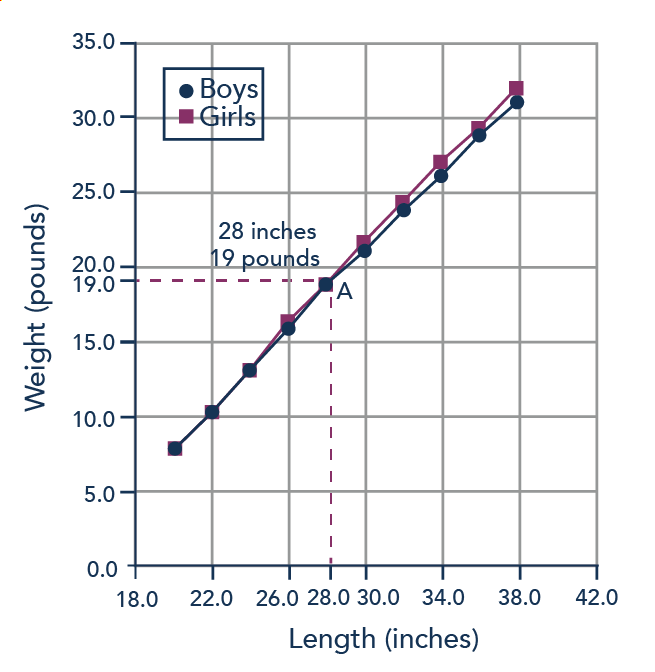Figure 1. The Length-Weight Relationship for American Boys and Girls.

Length-to-Weight Relationship for American Boys and Girls
Boys from Birth to 36 Months Girls from Birth to 36 Months
Length (inches) Weight (pounds) Length (inches) Weight (pounds)
20.0 8.0 20.0 7.9
22.0 10.5 22.0 10.5
24.0 13.5 24.0 13.2
26.0 16.4 26.0 16.0
28.0 19.0 28.0 18.8
30.0 21.8 30.0 21.2
32.0 24.3 32.0 24.0
34.0 27.0 34.0 26.2
36.0 29.3 36.0 28.9
38.0 32.0 38.0 31.3

### Try It

Not all relationships in economics are linear. Sometimes they are curves. Figure 2, below, presents another example of a line graph, representing the data from the table underneath. In this case, the line graph shows how thin the air becomes when you climb a mountain. The horizontal axis of the figure shows altitude, measured in meters above sea level. The vertical axis measures the density of the air at each altitude. Air density is measured by the weight of the air in a cubic meter of space (that is, a box measuring one meter in height, width, and depth). As the graph shows, air pressure is heaviest at ground level and becomes lighter as you climb. Figure 1 shows that a cubic meter of air at an altitude of 500 meters weighs approximately one kilogram (about 2.2 pounds). However, as the altitude increases, air density decreases. A cubic meter of air at the top of Mount Everest, at about 8,828 meters, would weigh only 0.023 kilograms. The thin air at high altitudes explains why many mountain climbers need to use oxygen tanks as they reach the top of a mountain.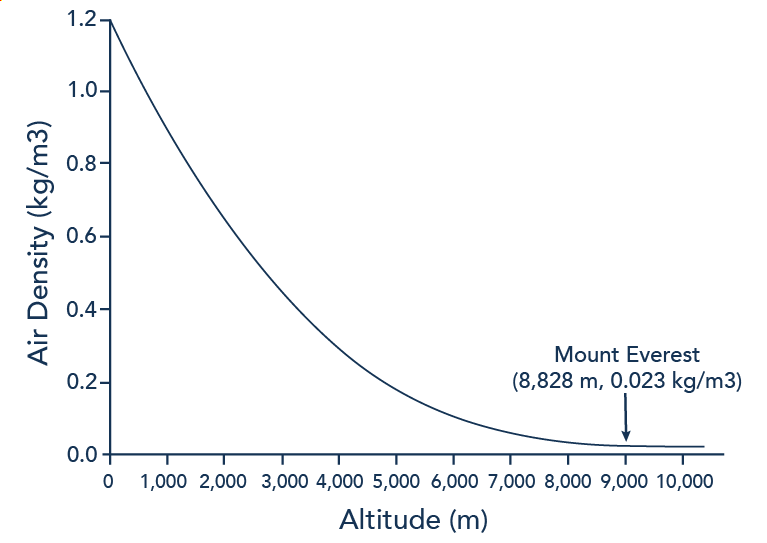Figure 2. Altitude–Air-Density Relationship.

Altitude–to–Air-Density Relationship
Altitude (meters) Air Density (kg/cubic meters)
0 1.200
500 1.093
1,000 0.831
1,500 0.678
2,000 0.569
2,500 0.484
3,000 0.415
3,500 0.357
4,000 0.307
4,500 0.231
5,000 0.182
5,500 0.142
6,000 0.100
6,500 0.085
7,000 0.066
7,500 0.051
8,000 0.041
8,500 0.025
9,000 0.022
9,500 0.019
10,000 0.014

The length-weight relationship and the altitude–air-density relationship in these two figures represent averages. If you were to collect actual data on air pressure at different altitudes, the same altitude in different geographic locations would have slightly different air density, depending on factors like how far you were from the equator, local weather conditions, and the humidity in the air. Similarly, in measuring the height and weight of children for the previous line graph, children of a particular height would have a range of different weights, some above average and some below. In the real world, this sort of variation in data is common. The task of a researcher is to organize that data in a way that helps to understand typical patterns. The study of statistics, especially when combined with computer statistics and spreadsheet programs, is a great help in organizing this kind of data, plotting line graphs, and looking for typical underlying relationships. For most economics and social science majors, a statistics course will be required at some point.

### Try It

One common line graph is called a time series, in which the horizontal axis shows time and the vertical axis displays another variable. Thus, a time-series graph shows how a variable changes over time. Figure 3 shows the unemployment rate in the United States since 1975, where unemployment is defined as the percentage of adults who want jobs and are looking for a job, but cannot find one. The points for the unemployment rate in each year are plotted on the graph, and a line then connects the points, showing how the unemployment rate has moved up and down since 1975. With a graph like this, it is easy to spot the times of high unemployment and of low unemployment.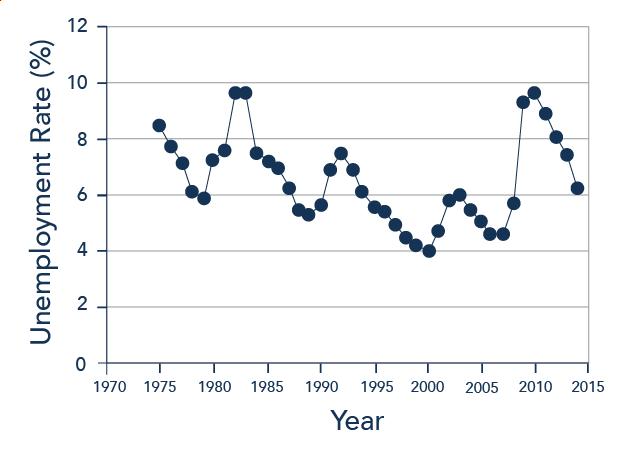Figure 3. U.S. Unemployment Rate, 1975–2014.

## Pie Graphs

A pie graph (sometimes called a pie chart) is used to show how an overall total is divided into parts. A circle represents a group as a whole. The slices of this circular “pie” show the relative sizes of subgroups.

Figure 4 shows how the U.S. population was divided among children, working-age adults, and the elderly in 1970, 2000, and what is projected for 2030. The information is first conveyed with numbers in the table, below, and then in three pie charts.

Table 3. U.S. Age Distribution, 1970, 2000, and 2030 (projected)
Year Total Population 19 and Under 20–64 years Over 65
1970 205.0 million 77.2 (37.6%) 107.7 (52.5%) 20.1 (9.8%)
2000 275.4 million 78.4 (28.5%) 162.2 (58.9%) 34.8 (12.6%)
2030 351.1 million 92.6 (26.4%) 188.2 (53.6%) 70.3 (20.0%)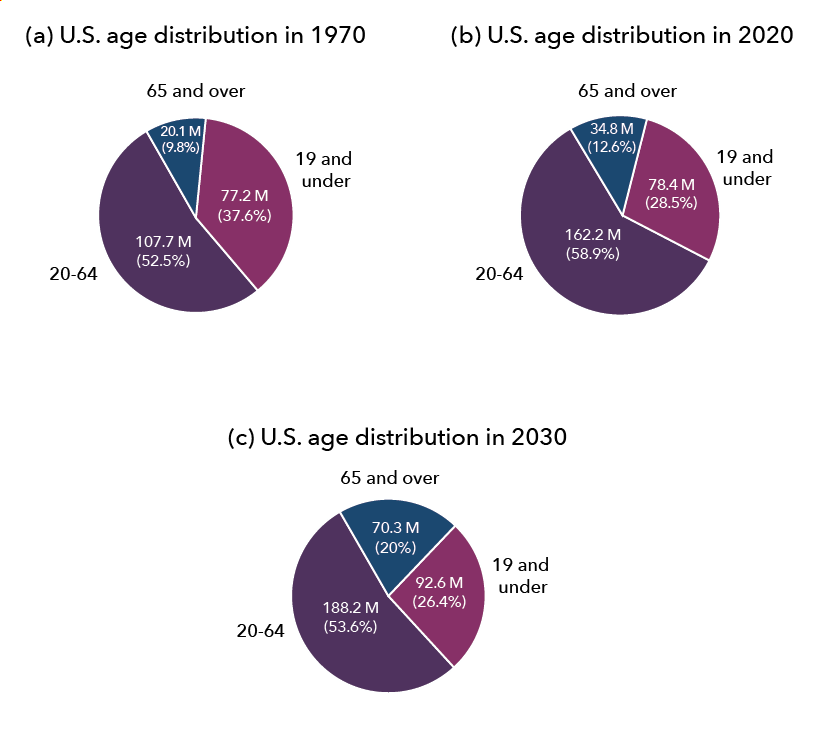Figure 4. Pie Graphs of the U.S. Age Distribution (numbers in millions).

In a pie graph, each slice of the pie represents a share of the total, or a percentage. For example, 50% would be half of the pie and 20% would be one-fifth of the pie. The three pie graphs in Figure 4 show that the share of the U.S. population 65 and over is growing. The pie graphs allow you to get a feel for the relative size of the different age groups from 1970 to 2000 to 2030, without requiring you to slog through the specific numbers and percentages in the table. Some common examples of how pie graphs are used include dividing the population into groups by age, income level, ethnicity, religion, occupation; dividing different firms into categories by size, industry, number of employees; and dividing up government spending or taxes into its main categories.

## Bar Graphs

A bar graph uses the height of different bars to compare quantities. The table, below, lists the 12 most populous countries in the world. Figure 5 provides this same data in a bar graph. The height of the bars corresponds to the population of each country. Although you may know that China and India are the most populous countries in the world, seeing how the bars on the graph tower over the other countries helps illustrate the magnitude of the difference between the sizes of national populations.

Table 4. Leading 12 Countries of the World by Population
Country Population
China 1,369
India 1,270
United States 321
Indonesia 255
Brazil 204
Pakistan 190
Nigeria 184
Russia 146
Japan 127
Mexico 121
Philippines 101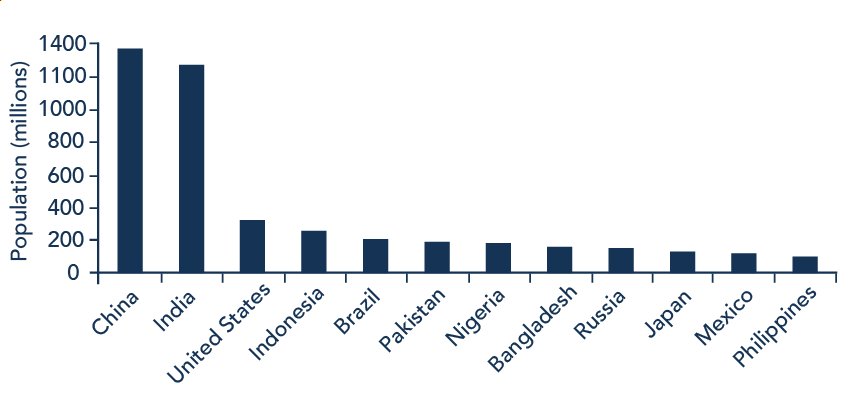Figure 5. The graph shows the 12 countries of the world with the largest populations. The height of the bars in the bar graph shows the size of the population for each country.

Bar graphs can be subdivided in a way that reveals information similar to that we can get from pie charts. Figure 6 offers three bar graphs based on the information from Figure 4 about the U.S. age distribution in 1970, 2000, and 2030. Figure 6 (a) shows three bars for each year, representing the total number of persons in each age bracket for each year. Figure 6 (b) shows just one bar for each year, but the different age groups are now shaded inside the bar. In Figure 6 (c), still based on the same data, the vertical axis measures percentages rather than the number of persons. In this case, all three bar graphs are the same height, representing 100 percent of the population, with each bar divided according to the percentage of population in each age group. It is sometimes easier for a reader to run his or her eyes across several bar graphs, comparing the shaded areas, rather than trying to compare several pie graphs.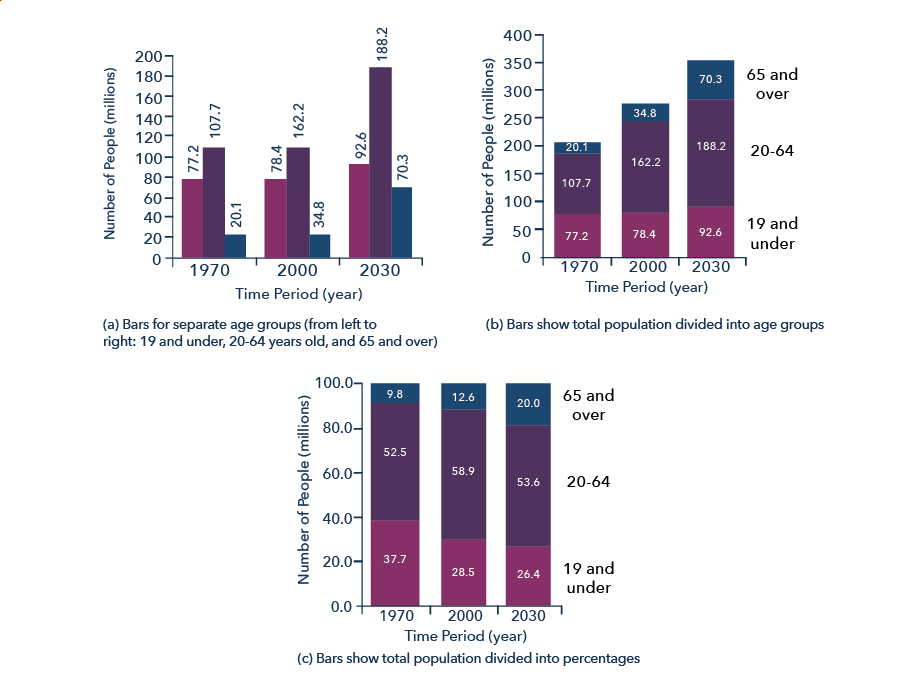Figure 6. U.S. Population with Bar Graphs.

Figure 5 and Figure 6 show how the bars can represent countries or years, and how the vertical axis can represent a numerical or a percentage value. Bar graphs can also compare size, quantity, rates, distances, and other quantitative categories.

## Comparing Line Graphs, Pie Charts, and Bar Graphs

Now that you are familiar with pie graphs, bar graphs, and line graphs, how do you know which graph to use for your data? Pie graphs are often better than line graphs at showing how an overall group is divided. However, if a pie graph has too many slices, it can become difficult to interpret.

Bar graphs are especially useful when comparing quantities. For example, if you are studying the populations of different countries, as in Figure 5, bar graphs can show the relationships between the population sizes of multiple countries. Not only can it show these relationships, but it can also show breakdowns of different groups within the population.

A line graph is often the most effective format for illustrating a relationship between two variables that are both changing. For example, time-series graphs can show patterns as time changes, like the unemployment rate over time. Line graphs are widely used in economics to present continuous data about prices, wages, quantities bought and sold, the size of the economy.

### Glossary

bar graph:
shows data as vertical bars; the height of different bars are used to compare quantities
line graph:
shows a relationship between two variables: one measured on the horizontal axis and the other measured on the vertical axis
pie graph:
used to show how an overall total is divided into parts. A circle represents a group as a whole and slices of this circular “pie” show the relative sizes of subgroups. Also called a pie chart
time series:
type of line graph in which the horizontal axis shows time and the vertical axis displays another variable. Thus, a time-series graph shows how a variable changes over time

## Contribute!

Did you have an idea for improving this content? We’d love your input.